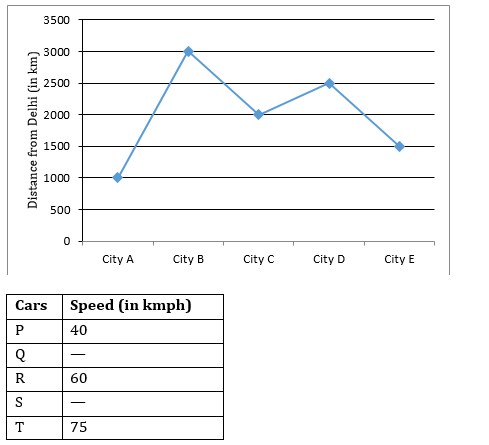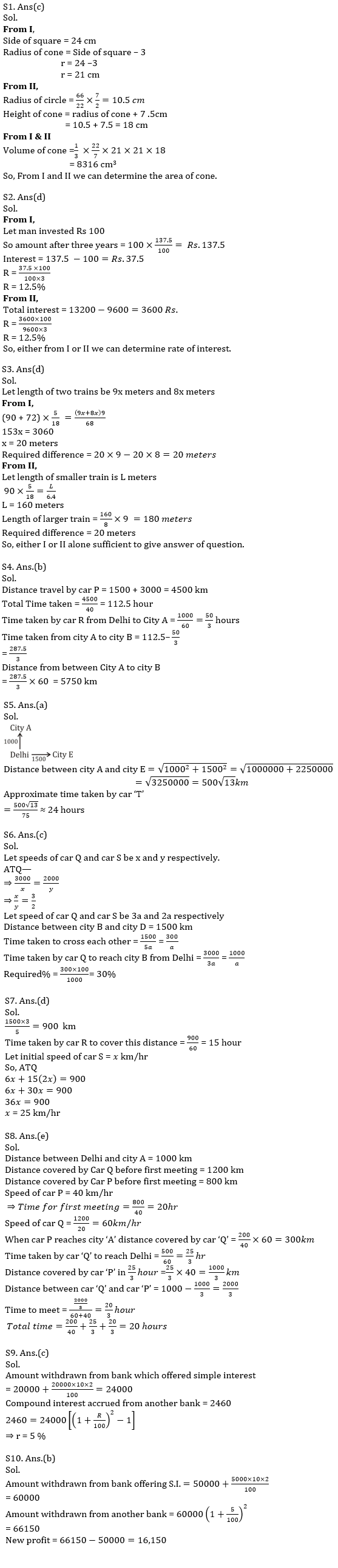Latest Banking jobs   »   Quantitative Aptitude Quiz For IBPS PO...

# Quantitative Aptitude Quiz For IBPS PO Mains 2022-15th January

Directions (1-3): The following questions are accompanied by two statements (I) and (II). You have to determine which statements(s) is/are sufficient/necessary to answer the questions.
(a) Statement (I) alone is sufficient to answer the question but statement (II) alone is not sufficient to answer the questions.
(b) Statement (II) alone is sufficient to answer the question but statement (I) alone is not sufficient to answer the question.
(c) Both the statements taken together are necessary to answer the questions, but neither of the statements alone is sufficient to answer the question.
(d) Either statement (I) or statement (II) by itself is sufficient to answer the question.
(e) Statements (I) and (II) taken together are not sufficient to answer the question.

Q1. What is volume of cone?
I . Radius of cone is 3 cm less than side of square, whose area is 576 cm2.
II . Height of cone is 7.5 cm more than radius of circle, which circumference is 66 cm.

Q2. What is rate of interest?
I . A man invested an amount for three years on simple interest and gets a total amount, which is 137.5% of invested amount.
II . Amir invested Rs. 9600 on simple interest and gets a total amount of Rs.13200 after three years.

Q3. The ratio between length of two trains is 9 : 8. What will be difference between lengths of both trains?
I . Speed of larger train and smaller train is 72 km/hr and 90 km/hr respectively. Both trains cross each other in 68/9 sec running in opposite direction.
II . Speed of smaller train is 90 km/hr and it cross a pole in 6.4 sec.

Directions (4-8): Line graph given below shows the distance between Delhi to five different cities in kilometer and Table given below shows the speed of five different cars in km/hrNOTE: – Some data is missing you have to calculate according to question.

Q4. Time taken by car ‘P’ to travel from city ‘E’ to Delhi and then Delhi to city ‘B’, is equal to the time taken by car ‘R’ to travel from Delhi to city ‘A’ and then city ‘A’ to city ‘B’. Find the distance between city ‘A’ and city ‘B’.
(a) 5650 km
(b) 5750 km
(c) 5450 km
(d) 5550 km
(e) 5320 km

Q5. Find the approximate time car ‘T’ takes to reach city ‘E’ from city ‘A’ if city ‘A’ and city ‘E’ is north and east direction of Delhi respectively.
(a) 24 hours
(b) 27 hours
(c) 20 hours
(d) 36 hours
(e) 42 hours

Q6. Car Q and Car S start from Delhi for city B and city C respectively and they reached in equal time. If Car Q and Car S starts from city B and city D respectively at same time and move towards each other, then time taken by car Q to cross car S is what percent of the time taken by car Q to reach city B from Delhi. Distance between city B and city D is 1500 km.
(a) 25%
(b) 20%
(c) 30%
(d) 40%
(e) 50%

Q7. A thief runs in a car S from Delhi to city E and after 6 hours of running, a policeman started to catch him in a car R. Due to this, thief increases the speed of his car by 100%. By this, the policeman is able to catch him at 3/5th of the distance of city E from Delhi. Find the initial speed of car ‘S’.
(a) 15 km/hr
(b) 27 km/hr
(c) 20 km/hr
(d) 25 km/hr
(e) 40 km/hr

Q8. Car P and Car Q start from Delhi for city A. Car Q first reaches at city A and meets car P in between the way, 200 km from city ‘A’. Find after how much time they will meet second time after first time meeting if they continue their to and fro motion.
(a) 24 hours
(b) 15 hours
(c) 16 hours
(d) 25 hours
(e) 20 hours

Directions (9-10): A person invested Rs. 20000 in a bank which is offering 10% per annum simple interest. After two years he withdrew the money from the bank and deposited the total amount in another bank which gives an interest rate of r% p.a. compounded annually. After 2 years he received an amount of Rs. 2460 more than what he had invested in that bank.

Q9. What is the value of r?
(a) 10%
(b) 15%
(c) 5%
(d) 12%
(e) None of these

Q10. If the person had invested Rs. 50,000 instead of 20000 in the bank that offered simple interest, what would have been his net profit after following the same procedure as given above?
(a) Rs. 16,800
(b) Rs. 16,150
(c) Rs. 16,350
(d) Rs. 16,000
(e) None of these

Solutions#### Congratulations!